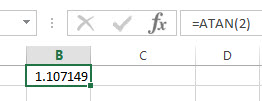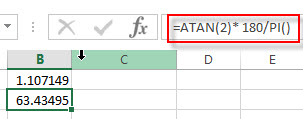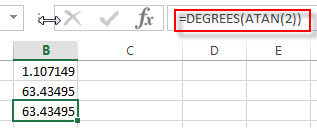# Excel ATAN Function

This post will guide you how to use Excel ATAN function with syntax and examples in Microsoft excel.

### Description

The Excel ATAN function returns the arctangent of a number. So this function can be used to calculates the arctangent of a supplied number. The returned angle is given in radians in the range –pi/2 to pi/2. Or the returned value is a numeric value.

The ATAN function is a build-in function in Microsoft Excel and it is categorized as a Math and Trigonometry Function.

The ATAN function is available in Excel 2016, Excel 2013, Excel 2010, Excel 2007, Excel 2003, Excel XP, Excel 2000, Excel 2011 for Mac.

### Syntax

The syntax of the ATAN function is as below:

`= ATAN (number)`

Where the ATAN function arguments is:

• number -This is a required argument. The tangent of the angle that you want to get.

Note:

• If you want to express the arctangent in degrees, you just need to multiply the result by 180/PI() or you can use the DEGREES function.
• If the supplied number is not a numeric value, the ATAN function will get the #VALUE！ Error。

### Excel ATAN Function Examples

The below examples will show you how to use Excel ATAN Function to return the arctangent for a given number.

1# get the arctangent of 2 in radians, pi/4, enter the following formula in Cell B1.

=ATAN(2)The returned result is 1.107149.

2# get the arctangent of 2 in degrees, using the following formula in Cell B2.

`=ATAN(2)* 180/PI()`This formula will return the value 63.43495.

Or you can use the below formula to achieve the same result:

`=DEGREES(ATAN(2))`### Related Functions

• Excel PI function
The Excel PI function returns the value of mathematical constant PI. The returned value is 3.14159265358979 and ti will accurate to 15 digits.The syntax of the PI function is as below:=PI()…
• Excel ATAN2 function
The Excel ATAN2 function returns the arctangent, or inverse tangent, of the specified x- and y-coordinates. And you can use the ATAN2 function to calculate the arctangent of a given x and y coordinates. The syntax of the ATAN2 function is as below:= ATAN2 (x_num, y_num)…
• Excel ATANH function
The Excel ATANH function returns the inverse hyperbolic tangent of a given number. The syntax of the ATANH function is as below:= ATANH (number)…
• Excel ACOS function
The Excel ACOS function returns the arccosine value of a number.The ACOS function is a build-in function in Microsoft Excel and it is categorized as a Math and Trigonometry Function.The syntax of the ACOS function is as below:= ACOS(number)…
• Excel ACOSH function
The Excel ACOSH function returns the inverse hyperbolic cosine of a number.The syntax of the ACOSH function is as below:= ACOSH (number)…
• Excel ASIN function
The Excel ASIN function returns the arcsine value of a number.The syntax of the ASIN function is as below:= ASIN (number)…
• Excel ASIN function
The Excel ATAN function returns the arctangent of a number. So this function can be used to calculates the arctangent of a supplied number. The returned angle is given in radians in the range –pi/2 to pi/2. The syntax of the ATAN function is as below:= ATAN (number)…
• Excel DEGREES Function
The Excel DEGREES function converts radians into degrees. And it will return a numeric value. The syntax of the DEGREES function is as below:=DEGREES (angle)…